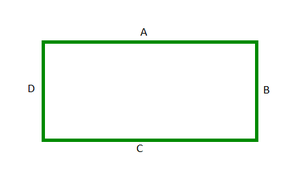# Java Program to Find the Perimeter of a Rectangle

A Rectangle is a quadrilateral with four right angles (90°). In a rectangle, the opposite sides are equal. A rectangle with all four sides equal is called a Square. A rectangle can also be called as right angled parallelogram.Rectangle

In the above rectangle, the sides A and C are equal and B and D are equal.

The perimeter of a rectangle is the total length of all its four sides. It can be simply calculated by all its four sides.

`Perimeter of rectangle ABCD = A+B+C+D`

Since the opposite sides are equal in a rectangle, it can be calculated as the sum of twice of one of its side and twice of its adjacent side.

`Perimeter of rectangle ABCD = 2A + 2B = 2(A+B)`

Program

## Java

 `// Java program to find the perimeter of a Rectangle`   `import` `java.io.*;`   `class` `GFG {` `  `  `    ``// Method to calculate the perimeter of the rectangle` `    ``// with given length and breadth` `    ``static` `void` `perimeter(``int` `length, ``int` `breadth)` `    ``{` `        ``// Calculate the 'perimeter' using the formula` `        ``int` `perimeter = ``2` `* (length + breadth);` `      `  `        ``System.out.println(``"The perimeter of the given rectangle of length "` `            ``+ length + ``" and breadth "` `+ breadth + ``" = "` `            ``+ perimeter);` `    ``}`   `    ``// Driver method` `    ``public` `static` `void` `main(String[] args)` `    ``{` `        ``// Initialize a variable length that stores length of` `        ``// the given rectangle` `        ``int` `length = ``10``;` `      `  `        ``// Initialize a variable breadth that stores breadth` `        ``// of the given rectangle` `        ``int` `breadth = ``20``;` `      `  `        ``// Call the perimeter method on these length and` `        ``// breadth` `        ``perimeter(length, breadth);` `    ``}` `}`

Output

`The perimeter of the given rectangle of length 10 and breadth 20 = 60`

Time complexity: O(1)

Auxiliary space: O(1)

Whether you're preparing for your first job interview or aiming to upskill in this ever-evolving tech landscape, GeeksforGeeks Courses are your key to success. We provide top-quality content at affordable prices, all geared towards accelerating your growth in a time-bound manner. Join the millions we've already empowered, and we're here to do the same for you. Don't miss out - check it out now!

Previous
Next